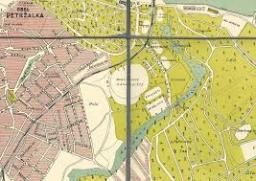# Represents 3509

What is the map's scale if the 2.5 cm long line represents 500 km?

M =  20000000

### Step-by-step explanation:

500•1000•100 = 2.5 • M

2.5M = 50000000

M = 20000000

Our simple equation calculator calculates it.Did you find an error or inaccuracy? Feel free to write us. Thank you!

Tips for related online calculators
Check out our ratio calculator.
Do you want to convert length units?